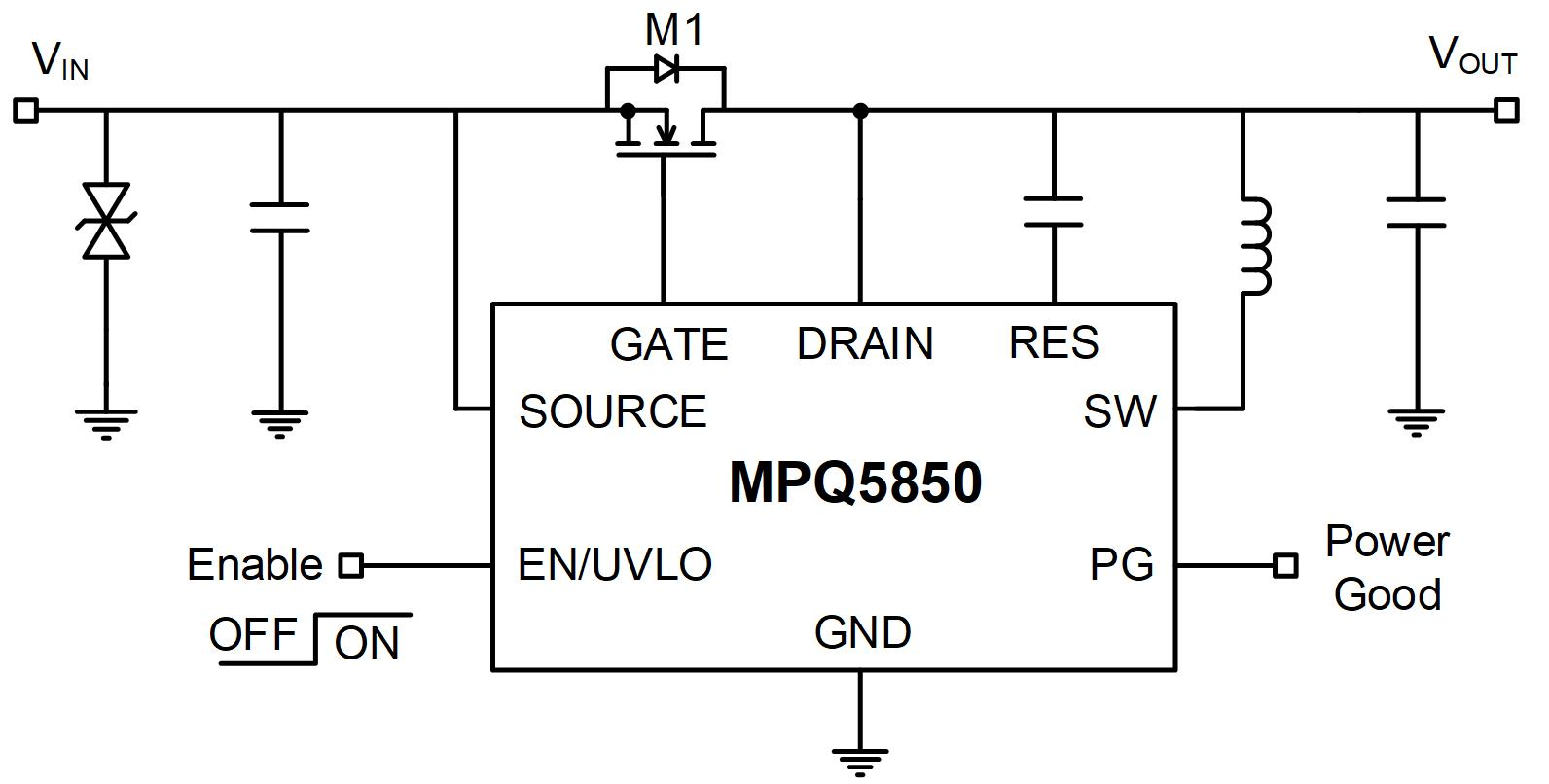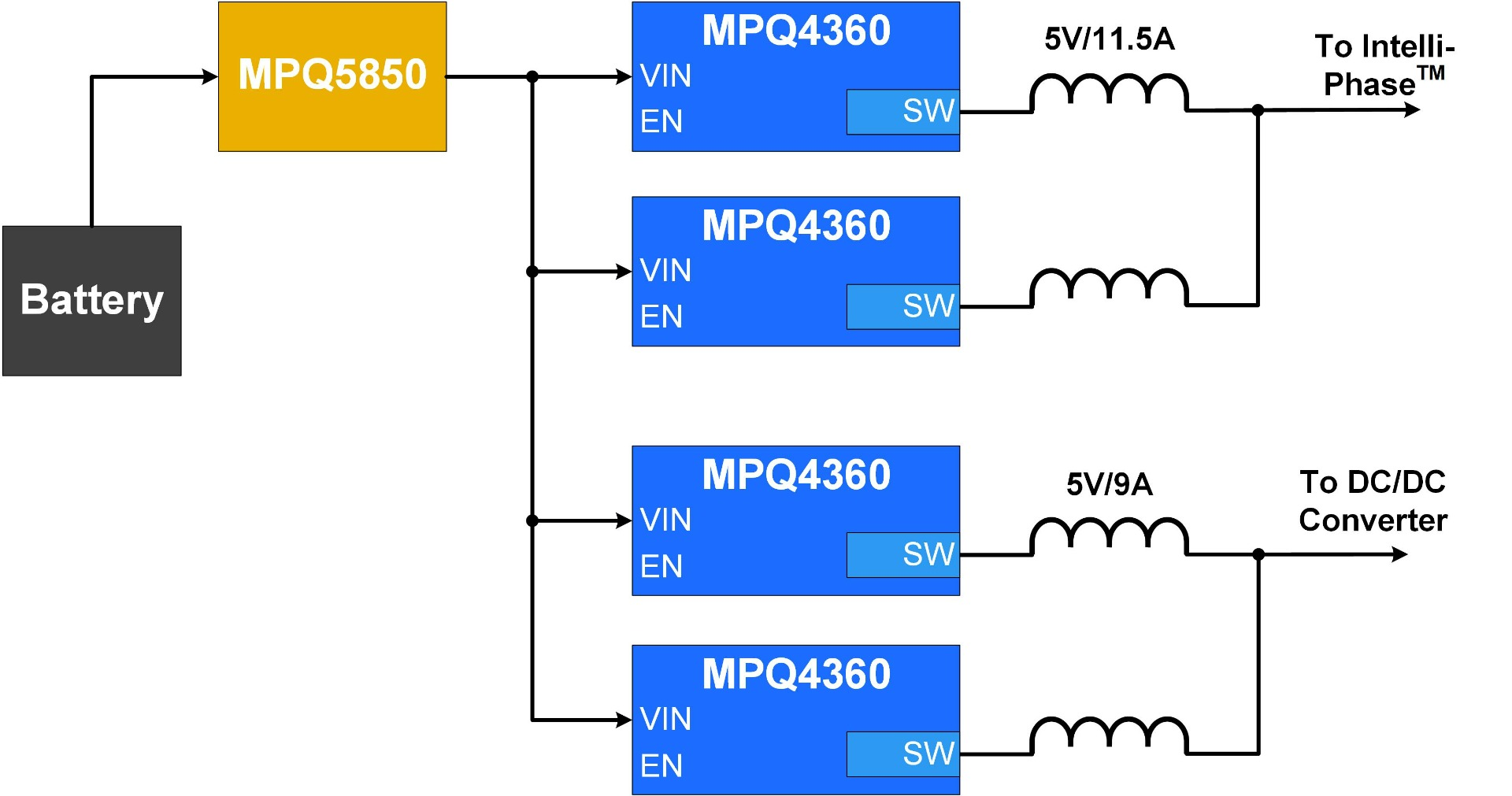# 汽车 SoC电源架构设计## 片上系统 (SoC)的电源要求

 电源轨名称 电压 (V) 电流 (C) 瞬态负载 (A) 斜率 (A/µs) 电压容差 (1)(%) 注意事项 VDD_CORE 0.85 60 40 40 3 1V8_GPIO 1.8 5 2.5 2.5 5 3V3_GPIO 3.3 5 2.5 2.5 5 1V8_analog 1.8 1.5 0.75 0.75 5 来自低噪声 DC/DC 变换器 DDR_VDD 1.05 6 3 3 3 DDR_VDDQ 0.6 6 3 3 5 PCIe 0.85 1.5 0.75 0.75 5 来自低噪声 DC/DC 变换器 MIPI 0.75 1.5 0.75 0.75 5 来自低噪声 DC/DC 变换器

1) 电压容差包括变换器的直流电压精度、负载瞬态响应和 IR 压降。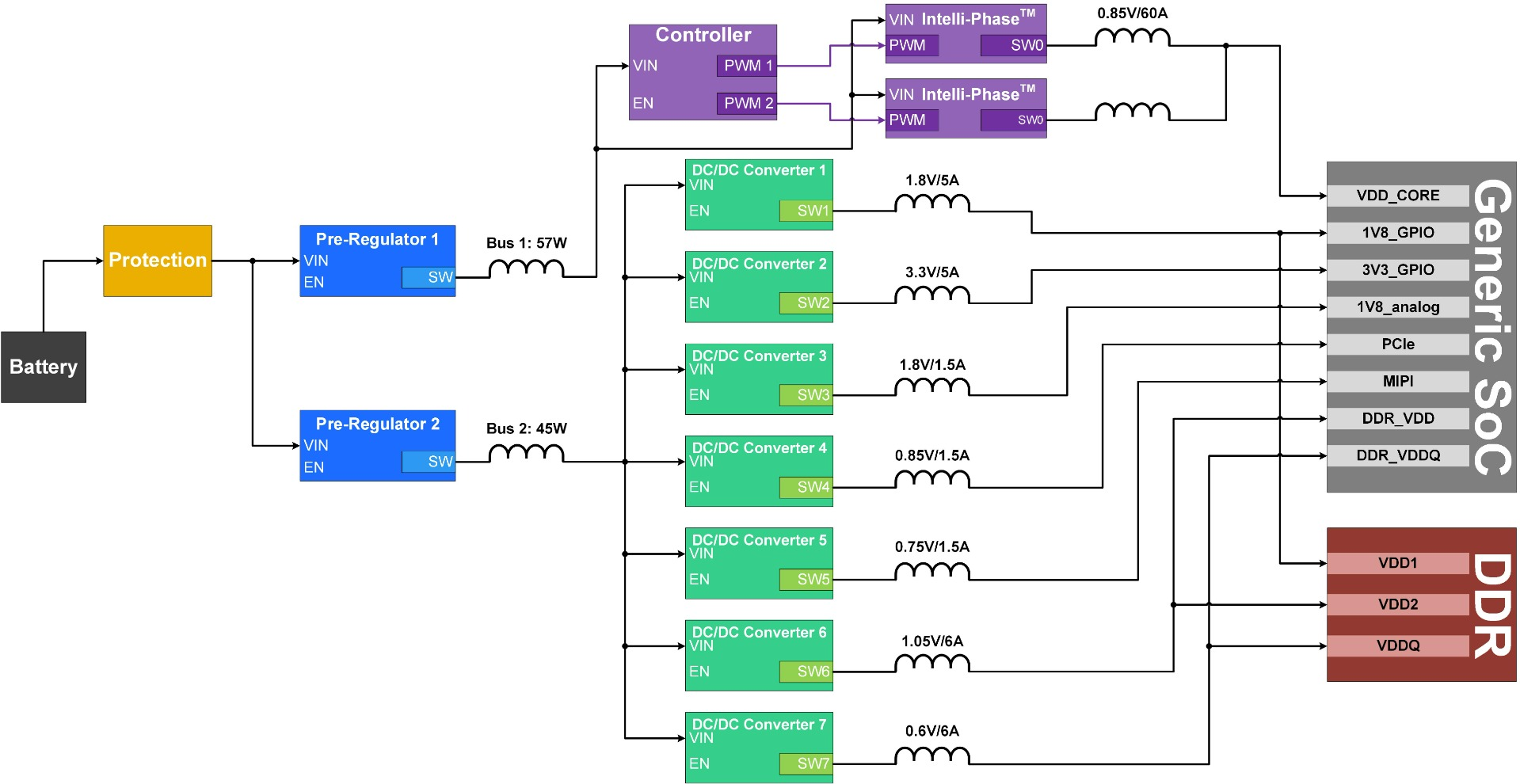## 设置总线电压

$$P_{PRE-REG1} = \frac {V \times I}{η} = \frac {0.85 \times 60}{0.89} = 57.3W$$

$$P_{PRE-REG2} = \frac {\sum_{1} ^{7}V_n \times l_n} {η} = \frac {1.8 \times 5+3.3 \times 5+1.8 \times 1.5+0.85 \times 1.5+0.75 \times 1.5+1.05 \times 6+0.6 \times 6}{0.89} = 45.5W$$

$$I_{PRE-REG1\_5V} = \frac {P}{V} = \frac {57.3}{5} = 11.5A$$

$$I_{PRE-REG2\_3.3V} = \frac {P}{V} = \frac {45.5}{3.3} = 13.8A$$

$$I_{PRE-REG2\_5} = \frac {P}{V} = \frac {45.5}{5} = 9.1A$$

## 选择IC

MPQ4360-AEC1 是一款额定电流为 6A 的同步降压变换器，它可在多相配置下工作以实现 12A 的输出电流。交错多相操作可减小电磁辐射并允许使用更小的组件；与采用控制器和分立 FET 的解决方案相比，它具有PCB 布局更小的优势。该器件还具有22µA超低静态电流(IQ)，因此非常适合汽车应用。图 2 显示了两个并联运行的 MPQ4360-AEC1。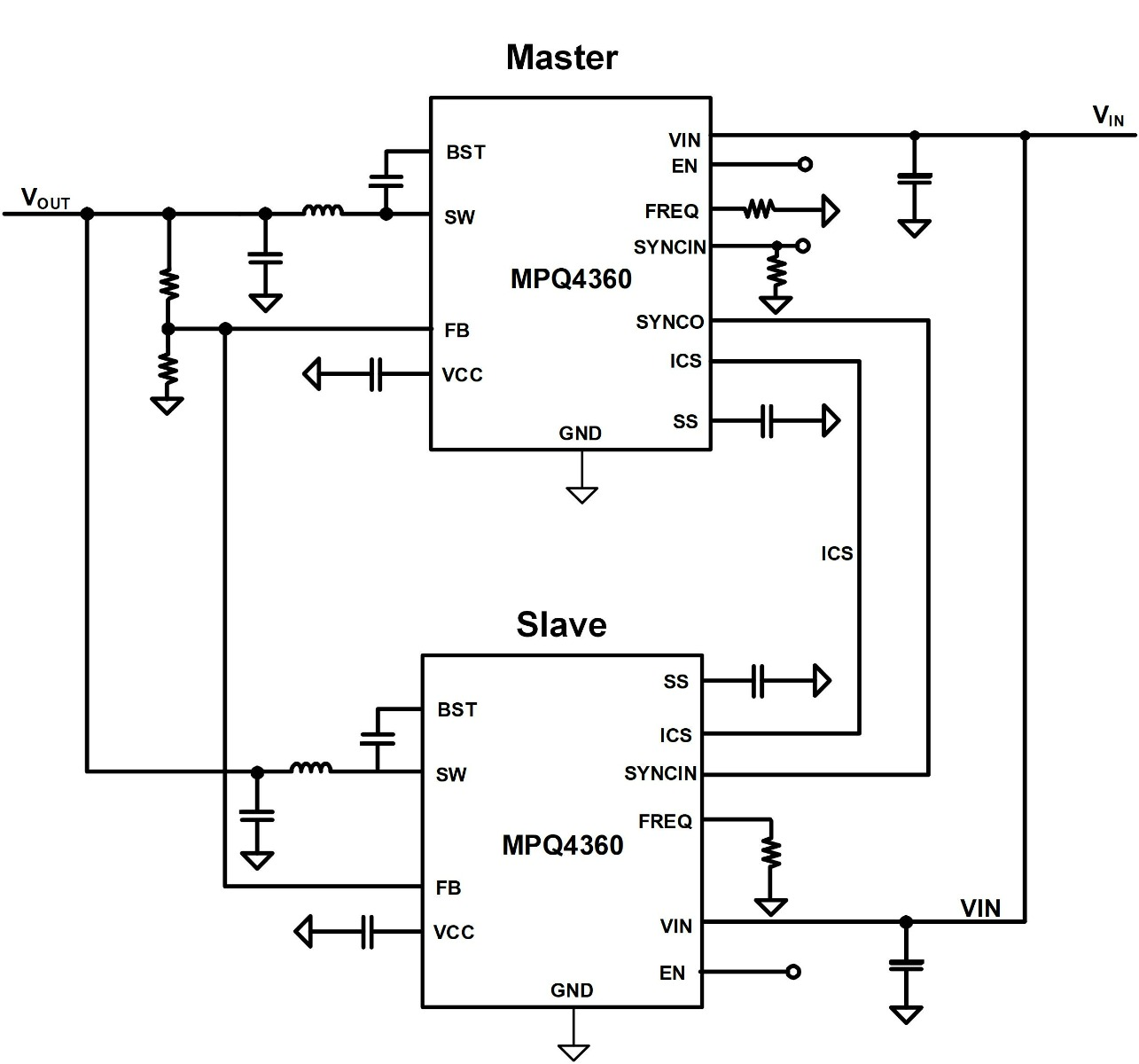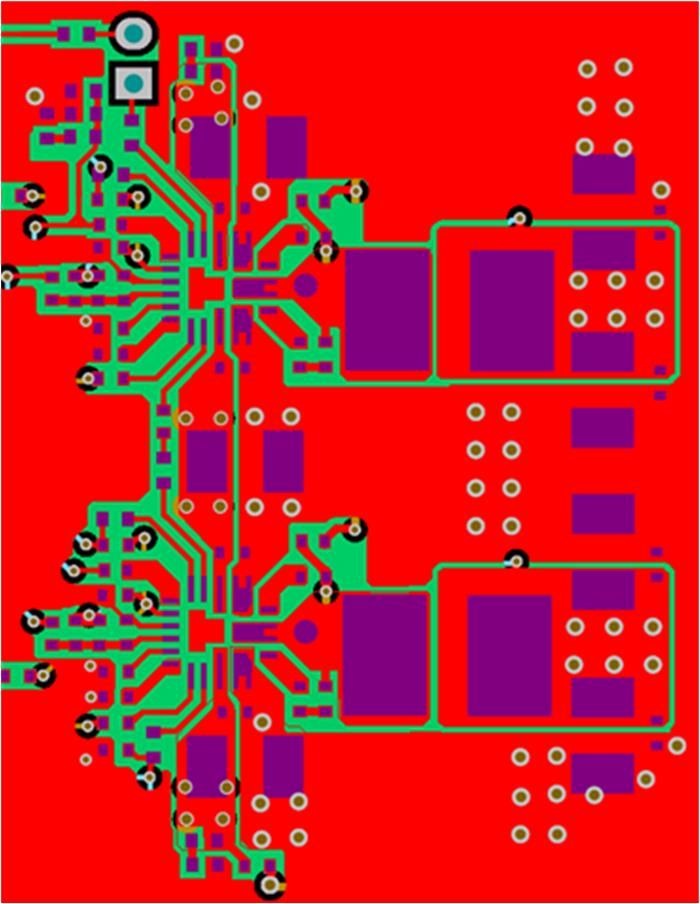## 系统保护

SoC系统的额定功率通常大于100W； 对于 一个12V 电池来说，这意味着输入电流可能超过 8A。而8A 对于一个简单的二极管来说显然过高；即使采用 VF 为 0.3V的肖特基二极管，功耗也会超过 2.4W。常用的替代方案是采用 P 沟道 MOSFET 来阻断反向电流，但这些 MOSFET 可能无法在足够长的时间内保护 IC 免受高频交流电流的影响。

MPQ5850-AEC1 是一款可以保护系统免受反向电流影响的理想二极管控制器。该器件控制一个具有强大栅极驱动能力的 N 沟道 MOSFET，可以快速阻断任何反向电流流动（见图 4），从而以最低功耗提供反向电流保护。